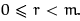Courses

# Principal Ideal Domain Mathematics Notes | EduRev

## Algebra for IIT JAM Mathematics

Created by: Veda Institute

## Mathematics : Principal Ideal Domain Mathematics Notes | EduRev

The document Principal Ideal Domain Mathematics Notes | EduRev is a part of the Mathematics Course Algebra for IIT JAM Mathematics.
All you need of Mathematics at this link: Mathematics

3.2 Principal Ideal Domains

Definition 3.2.1 A principal ideal domain (PID) is an integral domain in which every ideal is principal.

Lemma 3.2.2 Z is a PID.

NOTE: Showing that Z is a PID means showing that if I is an ideal of Z, then there is some integer n for which I consists of all the integer multiples of n.

Proof: Suppose that I  Z is an ideal. If I = {0} then I is the principal ideal generated by 0 and I is principal. If I ≠ {0} then I contains both positive and negative elements. Let m be the least positive element of I. We will show that I = (m).

Certainly (m)  I as I must contain all integer mulitples of m. On the other hand suppose a ∈ I. Then we can write

a = mq + r

where q ∈ Z andThen r = a - qm. Since a ∈ I and -qm ∈ I, this means r ∈ I. It follows that r = 0, otherwise we have a contradiction to the choice of m. Thus a = qm and a ∈ (m). We conclude I = (m).

Lemma 3.2.3 Let F be afield. Then the polynomial ring F[x] is a PID.

NOTE: Recall that F[x] has one important property in common with Z, namely a division algorithm. This is the key to showing that F[x] is a PID.

Proof: Let I  F[x] be an ideal. If I = {0} then I = (0) and I is principal. If I ≠ {0}, let f(x) be a polynomial of minimal degree m in I. Then (f(x))  I since every polynomial multiple of f(x) is in I.

We will show that I = (f (x)). To see this suppose g(x) ∈ I. Then

g(x) = f(x)q(x) + r(x)

where q(x),r(x) ∈ F[x] and r(x) = 0 or deg(r(x)) < m. Now

r(x) = g(x) — f(x)q(x)

and so r(x) ∈ I. It follows that r(x) = 0 otherwise r(x) is a polynomial in I of degree strictly less than m, contrary to the choice of f(x).

Thus g(x) = f(x)q(x), g(x) ∈ (f(x)) and I = (f(x)).

Question for the Seminar: If R is a ring (not a field) it is not always true that R[x] is a PID.

Find an example of a non-principal ideal in Z[x].

Offer running on EduRev: Apply code STAYHOME200 to get INR 200 off on our premium plan EduRev Infinity!

58 docs

,

,

,

,

,

,

,

,

,

,

,

,

,

,

,

,

,

,

,

,

,

;